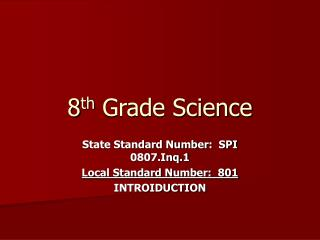DownloadDownload Presentation8 th Grade Science

# 8 th Grade Science

Download Presentation## 8 th Grade Science

- - - - - - - - - - - - - - - - - - - - - - - - - - - E N D - - - - - - - - - - - - - - - - - - - - - - - - - - -
##### Presentation Transcript

1. 8th Grade Science State Standard Number: SPI 0807.Inq.1 Local Standard Number: 801 INTROIDUCTION

2. Design a simple experimental procedure with an identified control and appropriate variables. Key vocabulary: hypothesis dependent variable experiment control factor variable control setup independent variable experimental setup

3. Design a simple experimental procedure with an identified control and appropriate variables. Key concepts/skills: Level 1: Define key vocabulary. Level 2: Given a hypothesis, identify independent and dependent variables. Level 3: Compare and contrast experimental setup and control setup (identify control factors.)

4. Design a simple experimental procedure with an identified control and appropriate variables. Steps in the Scientific Method (Review of Previous Learning) • Observation- • Question- • Hypothesis- • Testing- • Analysis- • Conclusion-

5. Design a simple experimental procedure with an identified control and appropriate variables. For this standard, we will concentrate on understanding steps three and four, “hypothesis” and “testing.”

6. Design a simple experimental procedure with an identified control and appropriate variables. Today we will practice this skill: • Analyzing the hypothesis to determine what the independent and dependent variables are.

7. Identify the variables in the hypothesis Example Hypothesis: Adding salt to water will raise the boiling point of the water. Independent variable - The variable that you, the scientist, will change on purpose to see what effect it has on the results of the experiment. Question: What is the independent variable in the above hypothesis? Answer: Adding salt is the independent variable because you can change whether you add salt to the water or not and how much salt you add to see how it changes the boiling point of the water.

8. Identify the variables in the hypothesis Example Hypothesis: Adding salt to water will raise the boiling point of the water. Dependent variable - The variable that depends on how you change the independent variable. It is the result of the test that you will record. Question: What is the dependent variable in the above hypothesis? Answer: The boiling point is the dependent variable because it depends on how you change the amount of salt in the water. It is the result that you will measure and record.

9. Practice Part 1 Identify the independent variable in each of following hypotheses: 1. Warmer temperatures decrease the hatching times for robin eggs. 2. Crickets chirp more when they get more food. 3. Dogs howl more when the moon is full. Answers: 1. temperature-you change the temperature in each test 2. amount of food-you change the amount of food in each test 3. amount of moonlight-you change the amount of moonlight in each test

10. Practice Part 2 Identify the dependent variable in each of following hypotheses: 1. Warmer temperatures decrease the hatching times for robin eggs. 2. Crickets chirp more when they get more food. 3. Dogs howl more when the moon is full. Answers: 1. hatching time-it depends on the temperature 2. amount of chirping-it depends on the food 3. amount of howling-it depends on the amount of moonlight